# Non Local Means¶

Important: Please read the installation page for details about how to install the toolboxes. $\newcommand{\dotp}{\langle #1, #2 \rangle}$ $\newcommand{\enscond}{\lbrace #1, #2 \rbrace}$ $\newcommand{\pd}{ \frac{ \partial #1}{\partial #2} }$ $\newcommand{\umin}{\underset{#1}{\min}\;}$ $\newcommand{\umax}{\underset{#1}{\max}\;}$ $\newcommand{\umin}{\underset{#1}{\min}\;}$ $\newcommand{\uargmin}{\underset{#1}{argmin}\;}$ $\newcommand{\norm}{\|#1\|}$ $\newcommand{\abs}{\left|#1\right|}$ $\newcommand{\choice}{ \left\{ \begin{array}{l} #1 \end{array} \right. }$ $\newcommand{\pa}{\left(#1\right)}$ $\newcommand{\diag}{{diag}\left( #1 \right)}$ $\newcommand{\qandq}{\quad\text{and}\quad}$ $\newcommand{\qwhereq}{\quad\text{where}\quad}$ $\newcommand{\qifq}{ \quad \text{if} \quad }$ $\newcommand{\qarrq}{ \quad \Longrightarrow \quad }$ $\newcommand{\ZZ}{\mathbb{Z}}$ $\newcommand{\CC}{\mathbb{C}}$ $\newcommand{\RR}{\mathbb{R}}$ $\newcommand{\EE}{\mathbb{E}}$ $\newcommand{\Zz}{\mathcal{Z}}$ $\newcommand{\Ww}{\mathcal{W}}$ $\newcommand{\Vv}{\mathcal{V}}$ $\newcommand{\Nn}{\mathcal{N}}$ $\newcommand{\NN}{\mathcal{N}}$ $\newcommand{\Hh}{\mathcal{H}}$ $\newcommand{\Bb}{\mathcal{B}}$ $\newcommand{\Ee}{\mathcal{E}}$ $\newcommand{\Cc}{\mathcal{C}}$ $\newcommand{\Gg}{\mathcal{G}}$ $\newcommand{\Ss}{\mathcal{S}}$ $\newcommand{\Pp}{\mathcal{P}}$ $\newcommand{\Ff}{\mathcal{F}}$ $\newcommand{\Xx}{\mathcal{X}}$ $\newcommand{\Mm}{\mathcal{M}}$ $\newcommand{\Ii}{\mathcal{I}}$ $\newcommand{\Dd}{\mathcal{D}}$ $\newcommand{\Ll}{\mathcal{L}}$ $\newcommand{\Tt}{\mathcal{T}}$ $\newcommand{\si}{\sigma}$ $\newcommand{\al}{\alpha}$ $\newcommand{\la}{\lambda}$ $\newcommand{\ga}{\gamma}$ $\newcommand{\Ga}{\Gamma}$ $\newcommand{\La}{\Lambda}$ $\newcommand{\si}{\sigma}$ $\newcommand{\Si}{\Sigma}$ $\newcommand{\be}{\beta}$ $\newcommand{\de}{\delta}$ $\newcommand{\De}{\Delta}$ $\newcommand{\phi}{\varphi}$ $\newcommand{\th}{\theta}$ $\newcommand{\om}{\omega}$ $\newcommand{\Om}{\Omega}$

This numerical tour study image denoising using non-local means. This algorithm has been introduced for denoising purposes in BuaCoMoA05

In :
addpath('toolbox_signal')

[Warning: Function isrow has the same name as a MATLAB builtin. We suggest you
rename the function to avoid a potential name conflict.]
[> In path at 110
In pymat_eval at 38
In matlabserver at 27]
[Warning: Function isrow has the same name as a MATLAB builtin. We suggest you
rename the function to avoid a potential name conflict.]
[> In path at 110
In pymat_eval at 38
In matlabserver at 27]


## Patches in Images¶

This numerical tour is dedicated to the study of the structure of patches in images.

Size $N = n \times n$ of the image.

In :
n = 128;


We load a noisy image $f_0\in \RR^N$.

In :
c = [100 200];
f0 = rescale( crop(f0,n, c) );


Display $f_0$.

In :
clf;
imageplot(f0);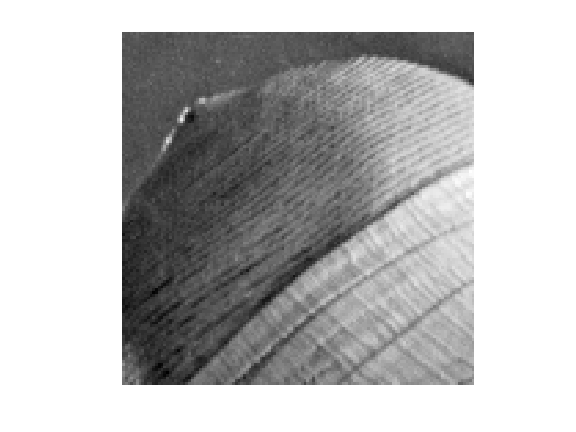Noise level $\si$.

In :
sigma = .04;


Generate a noisy image $f=f_0+\epsilon$ where $\epsilon \times \Nn(0,\si^2\text{Id}_N)$.

In :
f = f0 + randn(n,n)*sigma;


Display $f$.

In :
clf;
imageplot(clamp(f));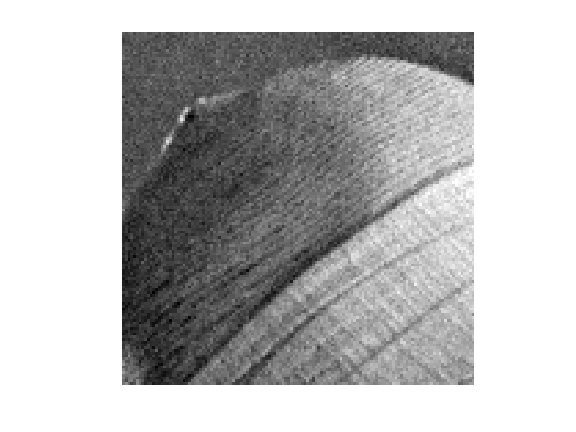We denote $w$ to be the half width of the patches, and $w_1=2w+1$ the full width.

In :
w = 3;
w1 = 2*w+1;


We set up large $(n,n,w_1,w_1)$ matrices to index the the X and Y position of the pixel to extract.

location of pixels

In :
[Y,X] = meshgrid(1:n,1:n);


offsets

In :
[dY,dX] = meshgrid(-w:w,-w:w);


location of pixels to extract

In :
dX = reshape(dX, [1 1 w1 w1]);
dY = reshape(dY, [1 1 w1 w1]);
X = repmat(X, [1 1 w1 w1]) + repmat(dX, [n n 1 1]);
Y = repmat(Y, [1 1 w1 w1]) + repmat(dY, [n n 1 1]);


We handle boundary condition by reflexion

In :
X(X<1) = 2-X(X<1); Y(Y<1) = 2-Y(Y<1);
X(X>n) = 2*n-X(X>n); Y(Y>n) = 2*n-Y(Y>n);


Patch extractor operator

In :
patch = @(f)f(X + (Y-1)*n);


Define the patch matrix $P$ of size $(n,n,w_1,w_1)$. Each |P(i,j,:,:)| represent an $(w_1,w_1)$ patch extracted around pixel $(i,j)$ in the image.

In :
P = patch(f);


Display some example of patches

In :
clf;
for i=1:16
x = floor( rand*(n-1)+1 );
y = floor( rand*(n-1)+1 );
imageplot( squeeze(P(x,y,:,:)), '', 4,4,i );
end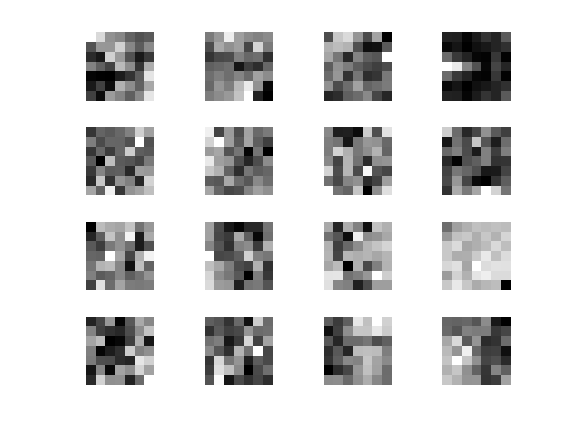## Dimensionality Reduction with PCA¶

Since NL-means type algorithms require the computation of many distances between patches, it is advantagous to reduce the dimensionality of the patch while keeping as much as possible of information.

Target dimensionality $d$.

In :
d = 25;


A linear dimensionality reduction is obtained by Principal Component Analysis (PCA) that projects the data on a small number of leading direction of the coveriance matrix of the patches.

Turn the patch matrix into an |(w1w1,nn)| array, so that each |P(:,i)| is a |w1*w1| vector representing a patch.

In :
resh = @(P)reshape(P, [n*n w1*w1])';


operator to remove the mean of the patches to each patch.

In :
remove_mean = @(Q)Q - repmat(mean(Q), [w1*w1 1]);


Compute the mean and the covariance of the points cloud representing the patches.

In :
P1 = remove_mean(resh(P));
C = P1*P1';


Extract the eigenvectors, sorted by decreasing amplitude

In :
[V,D] = eig(C); D = diag(D);
[D,I] = sort(D, 'descend'); V = V(:,I);


Display the decaying amplitude of the eigenvalues.

In :
clf;
plot(D); axis('tight');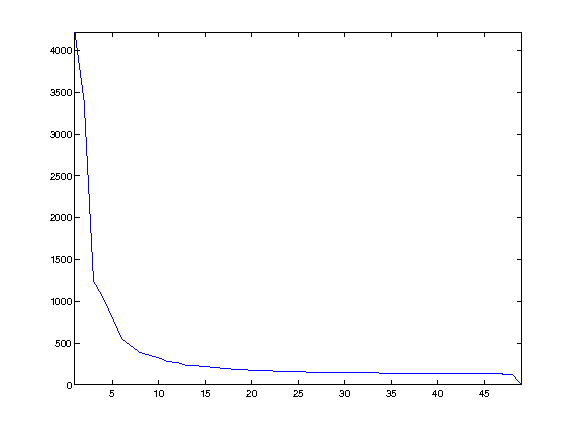Display the leading eigenvectors - they look like Fourier modes.

In :
clf;
for i=1:16
imageplot( reshape(V(:,i),[w1 w1]), '', 4,4,i );
end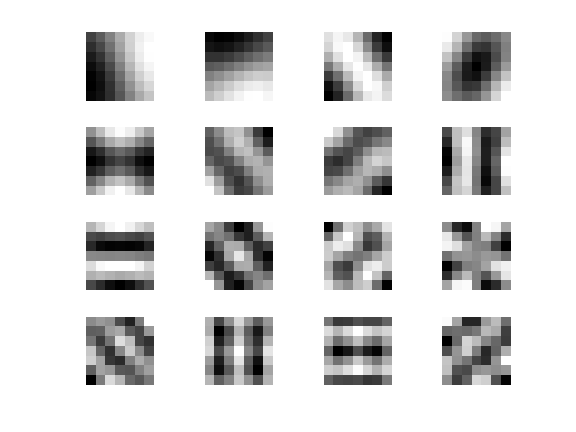Patch dimensionality reduction operator.

In :
iresh = @(Q)reshape(Q', [n n d]);
descriptor = @(f)iresh( V(:,1:d)' * remove_mean(resh(P)) );


Each |H(i,j,:)| is a $d$-dimensional descriptor of a patch.

In :
H = descriptor(f);


## Non-local Filter¶

NL-means applies, to each pixel location, an adaptive averaging kernel that is computed from patch distances.

We denote $H_{i} \in \RR^d$ the descriptor at pixel $i$. We define the distance matrix $$D_{i,j} = \frac{1}{w_1^2}\norm{H_i-H_j}^2.$$

Operator to compute the distances $(D_{i,j})_j$ between the patch around $i=(i_1,i_2)$ and all the other ones.

In :
distance = @(i)sum( (H - repmat(H(i(1),i(2),:), [n n 1])).^2, 3 )/(w1*w1);


The non-local mean filter compute a denoised image $\tilde f$ as $$\tilde f_i = \sum_j K_{i,j} f_j$$ where the weights $K$ are computed as $$K_{i,j} = \frac{ \tilde K_{i,j} }{ \sum_{j'} \tilde K_{i,j'} } \qandq \tilde K_{i,j} = e^{-\frac{D_{i,j}}{2\tau^2}} .$$

The width $\tau$ of the Gaussian is very important and should be adapted to match the noise level.

Compute and normalize the weight.

In :
normalize = @(K)K/sum(K(:));
kernel = @(i,tau)normalize( exp( -distance(i)/(2*tau^2) ) );


Compute a typical example of kernel for some pixel position $(x,y)$.

In :
tau = .05;
i = [83 72];
D = distance(i);
K = kernel(i,tau);


Display the squared distance and the kernel.

In :
clf;
imageplot(D, 'D', 1,2,1);
imageplot(K, 'K', 1,2,2);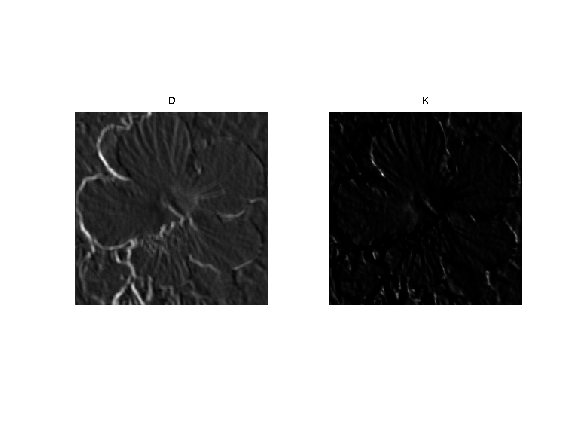## Localizing the Non-local Means¶

We set a "locality constant" $q$ that set the maximum distance between patches to compare. This allows to speed up computation, and makes NL-means type methods semi-global (to avoid searching in all the image).

In :
q = 14;


Using this locality constant, we compute the distance between patches only within a window. Once again, one should be careful about boundary conditions.

In :
selection = @(i){clamp(i(1)-q:i(1)+q, 1,n), clamp(i(2)-q:i(2)+q,1,n)};Compute distance and kernel only within the window.

In :
distance = @(i,sel)sum( (H(sel{1},sel{2},:) - repmat(H(i(1),i(2),:), ...
[length(sel{1}) length(sel{2}) 1])).^2, 3 )/(w1*w1);
distance = @(i)distance(i,selection(i));
kernel = @(i,tau)normalize( exp( -distance(i)/(2*tau^2) ) );


Compute a typical example of kernel for some pixel position $(x,y)$.

In :
D = distance(i);
K = kernel(i,tau);

---------------------------------------------------------------------------
RuntimeError                              Traceback (most recent call last)
<ipython-input-36-e26e7e6e5cea> in <module>()
----> 1 get_ipython().run_cell_magic('matlab', '', 'K = kernel(i,tau);')

/Users/gpeyre/anaconda/envs/py3k/lib/python3.3/site-packages/IPython/core/interactiveshell.py in run_cell_magic(self, magic_name, line, cell)
2160             magic_arg_s = self.var_expand(line, stack_depth)
2161             with self.builtin_trap:
-> 2162                 result = fn(magic_arg_s, cell)
2163             return result
2164

/Users/gpeyre/anaconda/envs/py3k/lib/python3.3/site-packages/pymatbridge/matlab_magic.py in matlab(self, line, cell, local_ns)

/Users/gpeyre/anaconda/envs/py3k/lib/python3.3/site-packages/IPython/core/magic.py in <lambda>(f, *a, **k)
191     # but it's overkill for just that one bit of state.
192     def magic_deco(arg):
--> 193         call = lambda f, *a, **k: f(*a, **k)
194
195         if callable(arg):

/Users/gpeyre/anaconda/envs/py3k/lib/python3.3/site-packages/pymatbridge/matlab_magic.py in matlab(self, line, cell, local_ns)
215             e_s += "\n-----------------------"
216             e_s += "\nAre you sure Matlab is started?"
--> 217             raise RuntimeError(e_s)
218
219

RuntimeError: There was an error running the code:
K = kernel(i,tau);
-----------------------
Are you sure Matlab is started?

Display the squared distance and the kernel.

In [ ]:
clf;
imageplot(D, 'D', 1,2,1);
imageplot(K, 'K', 1,2,2);


The NL-filtered value at pixel $(x,y)$ is obtained by averaging the values of $f$ with the weight $K$.

In [ ]:
NLval = @(K,sel)sum(sum(K.*f(sel{1},sel{2})));
NLval = @(i,tau)NLval( kernel(i,tau), selection(i) );


We apply the filter to each pixel location to perform the NL-means algorithm.

In [ ]:
[Y,X] = meshgrid(1:n,1:n);
NLmeans = @(tau)arrayfun(@(i1,i2)NLval([i1 i2],tau), X,Y);


Display the result for some value of $\tau$.

In [ ]:
tau = .03;
clf;
imageplot(NLmeans(tau));


Exercise 1

Compute the denoising result for several values of $\tau$ in order to determine the optimal denoising that minimizes $\norm{\tilde f - f_0}$.

In [ ]:
exo1()

In [ ]:
%% Insert your code here.


Display the best result.

In [ ]:
clf;
imageplot(clamp(fNL));


Exercise 2

Explore the influence of the $q$ and $w$ parameters.

In [ ]:
exo2()

In [ ]:
%% Insert your code here.